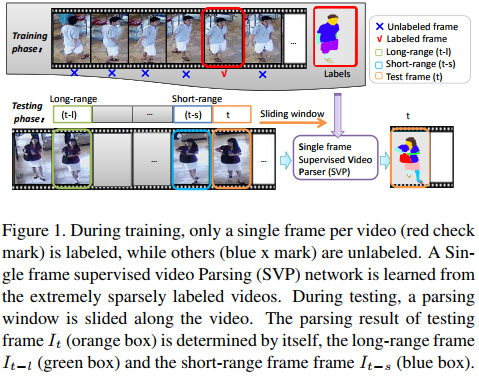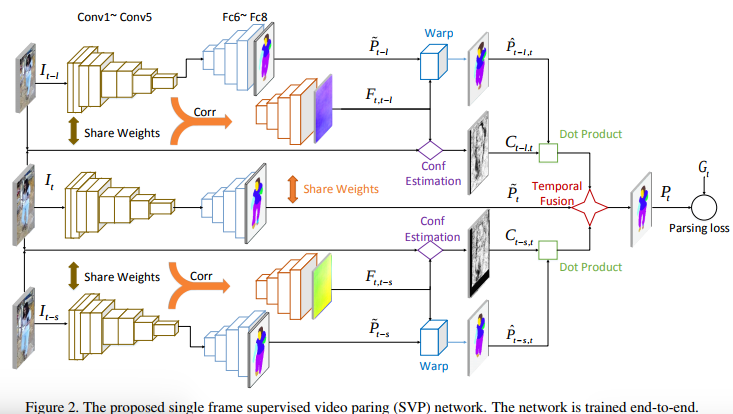## Summary

The goal is to segment video frames into several labels, e.g., face, pants, left-leg. In this paper, they develop a method called Single frame Video Parsing (SVP) which requires only one labeled frame per video in training stage. SVP (i) roughly parses the frames within the video segment, (ii) estimates the optical flow between frames and (iii) fuses the rough parsing results warped by optical flow to produce the refined parsing results.### Architecture

The architecture is described below$$I_{t}, I_{t-l}, I_{t-s}$$ are input frames and only $$I_{t}$$ has a ground truth

$$F_{t,t-s}, F_{t,t-l}$$ are the estimated optical flow fields

$$P_{t-s,t}, P_{t-l,t}$$ are the estimated segmentation maps

$$C_{t-s,t}, C_{t-l,t}$$ are optical flow confidense maps

$$\hat{P}_{t},\hat{P}_{t-s}, \hat{P}_{t-l}$$ are the segmentation maps without optical flow refinement

$$P_{t}$$ is the final segmentation map

## Experiments and ResultsThe main limitation of this method is that it only works on small videos.